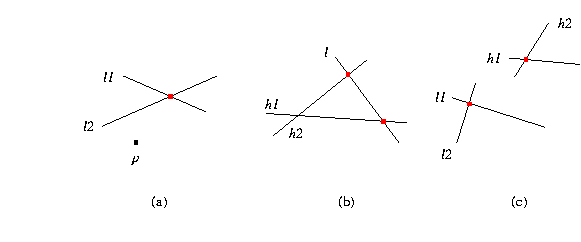## CGAL::compare_x

Comparison_result compare_x ( Point_2<Kernel> p, Point_2<Kernel> q)
compares the x-coordinates of p and q.

Comparison_result compare_x ( Point_3<Kernel> p, Point_3<Kernel> q)
compares the x-coordinates of p and q.

Comparison_result
 compare_x ( Point_2 p, Line_2 l1, Line_2 l2)
compares the x-coordinates of p and the intersection of lines l1 and l2, see (a) in the figure below.

Comparison_result
 compare_x ( Line_2 l, Line_2 h1, Line_2 h2)
compares the x-coordinates of the intersection of line l with line h1 and with line h2, see (b) in the figure below.

Comparison_result
 compare_x ( Line_2 l1, Line_2 l2, Line_2 h1, Line_2 h2)
compares the x-coordinates of the intersection of lines l1 and l2 and the intersection of lines h1 and h2, see (c) in the figure below.